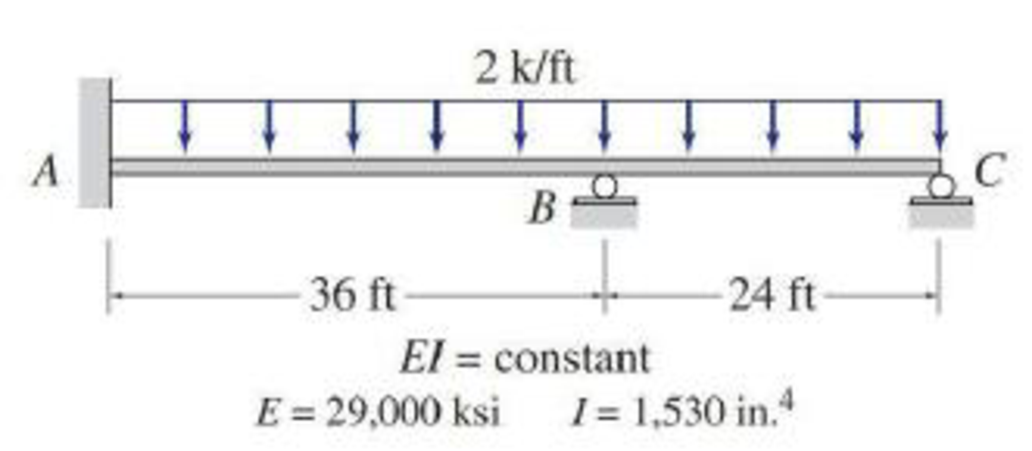# Determine the reactions and draw the shear and bending moment diagrams for the beams shown in Figs. P15.1–P15.5 by using the slope-deflection method. FIG. P15.4, P15.7

#### Solutions

Chapter
Section
Chapter 15, Problem 4P
Textbook Problem
298 views

## Determine the reactions and draw the shear and bending moment diagrams for the beams shown in Figs. P15.1–P15.5 by using the slope-deflection method.FIG. P15.4, P15.7

To determine

Find the reaction and plot the shear and bending moment diagram.

### Explanation of Solution

Fixed end moment:

Formula to calculate the fixed moment for point load with equal length are PL8.

Formula to calculate the fixed moment for point load with unequal length are Pab2L2 and Pa2bL2.

Formula to calculate the fixed moment for UDL is WL212.

Calculation:

Consider the flexural rigidity EI of the beam is constant.

Show the free body diagram of the entire beam as in Figure 1.

Refer Figure (1),

Calculate the fixed end moment for AB.

FEMAB=2×36212=216kft

Calculate the fixed end moment for BA.

FEMBA=2×36212=216kft

Calculate the fixed end moment for BC.

FEMBC=2×24212=96kft

Calculate the fixed end moment for CB.

FEMCB=2×24212=96kft

Calculate the slope deflection equation for the member AB.

MAB=2EIL(2θA+θB3ψ)+FEMAB

Here, θA is the slope at the point A and θB is the slope at the point B.

Substitute 29,000ksi for E, 1530in.4 for I, 0 for ψ, 0 for θA, 36ft for L and 216kft for FEMAB.

MAB=2×29,000×1,53036×144(2(0)+θB3(0))+216=17118.0556θB+216 (1)

Calculate the slope deflection equation for the member BA.

MBA=2EIL(2θB+θA3ψ)+FEMBA

Substitute 29,000ksi for E, 1530in.4 for I, 0 for ψ, 0 for θA, 36ft for L, and 216kft for FEMBA.

MBA=2×29,000×1,53036×144(2θB+03(0))216=34,236.11θB216 (2)

Calculate the slope deflection equation for the member BC.

MBC=3EIL(θB+θCψ)+FEMBC+FEMBC2

Substitute 29,000ksi for E, 1530in.4 for I, 0 for ψ, 0 for θC, 24ft for L and 96kft for FEMBC.

MBC=3×29,000×1,53024×144(θB+0(0))+96+962=38,515.625θB+144 (3)

Calculate the slope deflection equation for the member CB.

MCB=0

Write the equilibrium equation as below.

MBA+MBC=0

Substitute equation (1) and equation (2) in above equation.

34,236.11θB216+38,515.625θB+144=072,751.732θB72=0θB=7272,751.732θB=0.00099kft2

Substitute 0.00099kft2 for θB in equation (1).

MAB=17,118.0556(0.00099)+216=232.9kft

Substitute 0.000734kft2 for θB in equation (2).

MBA=34,236.11(0.00099)216=182.1kft

Substitute 0.000734kft2 for θB in equation (3).

MBC=38,515.625(0.00099)+144=182.1kft

Consider the member AB of the beam:

Show the section free body diagram of the member AB and BC as in Figure 2.

### Still sussing out bartleby?

Check out a sample textbook solution.

See a sample solution

#### The Solution to Your Study Problems

Bartleby provides explanations to thousands of textbook problems written by our experts, many with advanced degrees!

Get Started

Find more solutions based on key concepts
What is atmospheric pressure?

Engineering Fundamentals: An Introduction to Engineering (MindTap Course List)

List features of e-book readers and wearable devices.

Enhanced Discovering Computers 2017 (Shelly Cashman Series) (MindTap Course List)

What is competitive disadvantage? Why has it emerged as a factor?

Principles of Information Security (MindTap Course List)

Name two types of friction facings.

Automotive Technology: A Systems Approach (MindTap Course List)

If your motherboard supports ECC DDR3 memory, can you substitute non-ECC DDR3 memory?

A+ Guide to Hardware (Standalone Book) (MindTap Course List)

What are the best ways to remove slag between filler weld passes?

Welding: Principles and Applications (MindTap Course List)